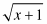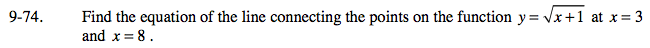### Home > PC > Chapter 9 > Lesson 9.2.1 > Problem9-74

9-74.

Find the equation of the line connecting the points on the function y =at x = 3 and x = 8. Homework Help ✎Determine the y-coordinates for each of the points.

Find the slope between the two points.

Choose one of the points and use the slope.
Write the equation of the line in point-slope form.
(yy1) = m(xx1)